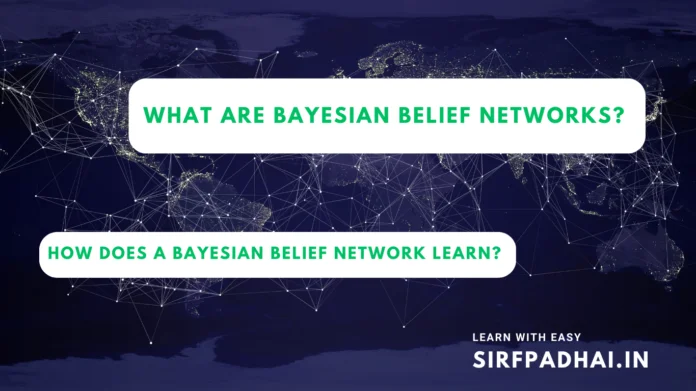# What are Bayesian belief networks? |How does a Bayesian belief network learn?# What are Bayesian belief networks?

Bayesian belief networks specify joint conditional probability distributions. They allow class conditional independencies to be defined between subsets of variables. They provide a graphical model of causal relationships, on which learning can be performed. Trained Bayesian belief networks can be used for classification. Bayesian belief networks are also known as belief networks, Bayesian networks, and probabilistic networks.

## How does a Bayesian belief network learn?

A belief network is defined by two components – a directed acyclic graph and a set of conditional probability tables. Each node in the directed acyclic graph represents a random variable. The variables may be discrete or continuously valued. They may correspond to actual attributes given in the data or to “hidden variables” believed to form a relationship. Each arc represents a probabilistic dependence. If an arc is drawn from a node Y to a node Z, then Y is a parent or immediate predecessor of Z and Z is a descendant of Y. Each variable is conditionally independent of its nondescendants in the graph, given its parents.

A belief network has one conditional probability table (CPT) for each variable. The CPT for variable Y specifies the conditional distribution P(Y |Parents (Y)), where Parents(Y) are the parents of Y.

Let X = (x1,…,xn) be a data tuple described by the variables or attributes Y1,….., Yn, respectively. Each variable is conditionally independent of its nondescendants in the network graph, given its parents. This allows the network to provide a complete representation of the existing joint probability distribution with the following equation –

where P( x1,…,xn ) is the probability of a particular combination of values of X, and the values for P(xi|Parents(Yi)) correspond to the entries in the CPT for Yi

A node within the network can be selected as an “output” node, representing a class label attribute. There may be more than one output node. Various algorithms for learning can be applied to the network.

In the learning or training of a belief network, a number of scenarios are possible. The network topology may be given in advance or inferred from the data. The network variables may be observable or hidden in all or some of the training tuples. The case of hidden data is also referred to as missing values or incomplete data.

Several algorithms exist for learning the network topology from the training data given observable variables. If the network topology is known and the variables are observable, then training the network is straightforward. It consists of computing the CPT entries, as is similarly done when computing the probabilities involved in naive Bayesian classification. When the network topology is given and some of the variables are hidden, there are various methods to choose from for training the belief network.

Let D be a training set of data tuples, X1,X2,……., X|D|. Training the belief network means that we must learn the values of the CPT entries. Let wijk be a CPT entry for the variable Yi=yij having the parents Ui=uik, where wijk = P(Yi=yij|Ui=uik) The wijk are viewed as weights, analogous to the weights in hidden units of neural networks. The set of weights is collectively referred to as W. The weights are initialized to random probability values. A gradient descent strategy performs greedy hill-climbing. At each iteration, the weights are updated and will eventually converge to a local optimum solution.

A gradient descent strategy is used to search for the wijk values that best model the data, based on the assumption that each possible setting of wijk is equally likely. Such a strategy is iterative. It searches for a solution along the negative gradient of a criterion function. We want to find the set of weights, W, that maximize this function. To start with, the weights are initialized to random probability values. The gradient descent method performs greedy hill-climbing in that, at each iteration or step along the way, the algorithm moves toward what appears to be the best solution at the moment, without backtracking. The weights are updated at each iteration. Eventually, they converge to a local optimum solution.